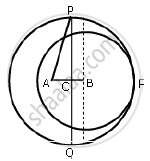Share

# Two Circle with Centres a and B, and Radii 5 Cm and 3 Cm, Touch Each Other Internally. If the Perpendicular Bisector of the Segment Ab Meets the Bigger Circle in P and Q; Find the Length of Pq. - Mathematics

Course

#### Question

Two circle with centres A and B, and radii 5 cm and 3 cm, touch each other internally. If the perpendicular bisector of the segment AB meets the bigger circle in P and Q; find the length of PQ.

#### SolutionIf two circles touch internally, then distance between their centres is equal to the difference of their radii.

So, AB = (5 − 3) cm = 2 cm.

Also, the common chord PQ is the perpendicular bisector of AB. Therefore, AC = CB =1/2 "AB"
= 1 cm

In right ΔACP, we have"AP"^2 = "AC"^2 + "CP"^2

⇒ 5^2 = 1^2 + "CP"^2

⇒ "CP"^2 = 25 - 1 = 24

⇒ "CP" = sqrt(24)= 2 sqrt(6)  "cm"

Now , PQ = 2 CP

= 2xx 2 sqrt(6)  "cm"

= 4 sqrt (6 )  "cm"

Is there an error in this question or solution?